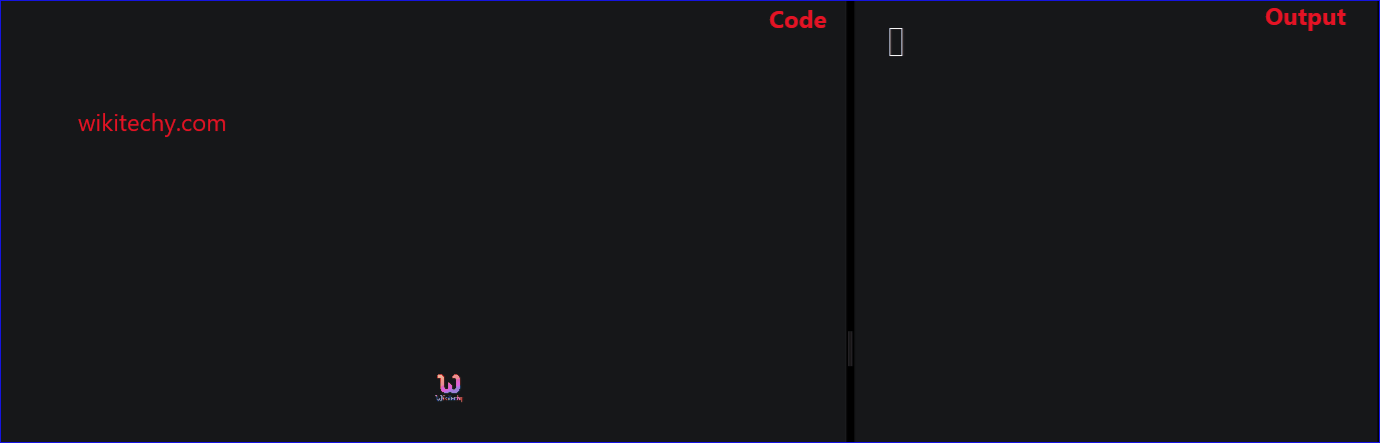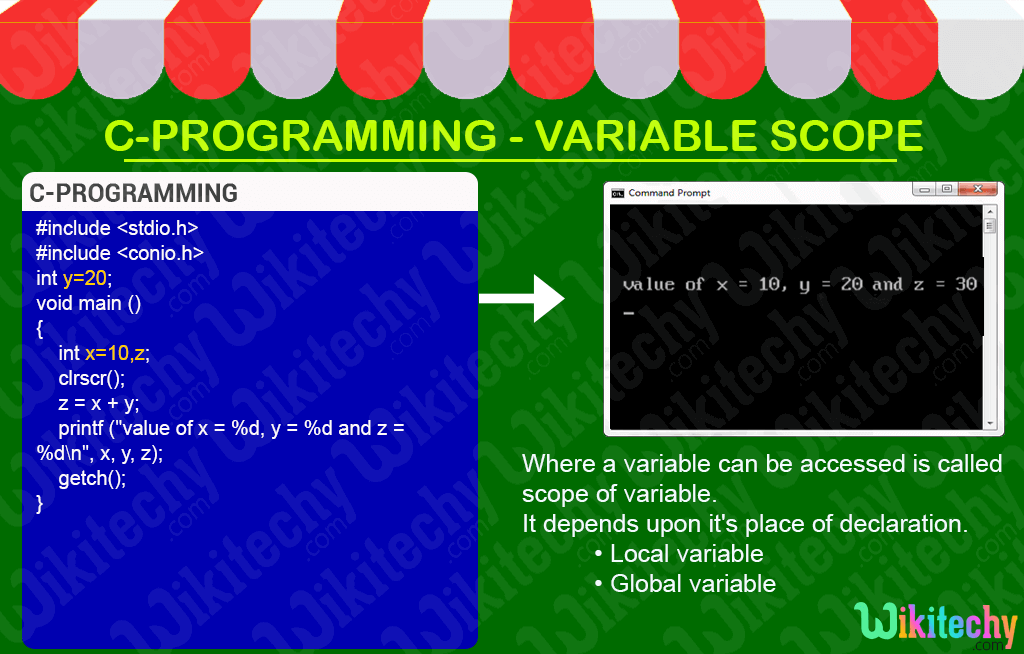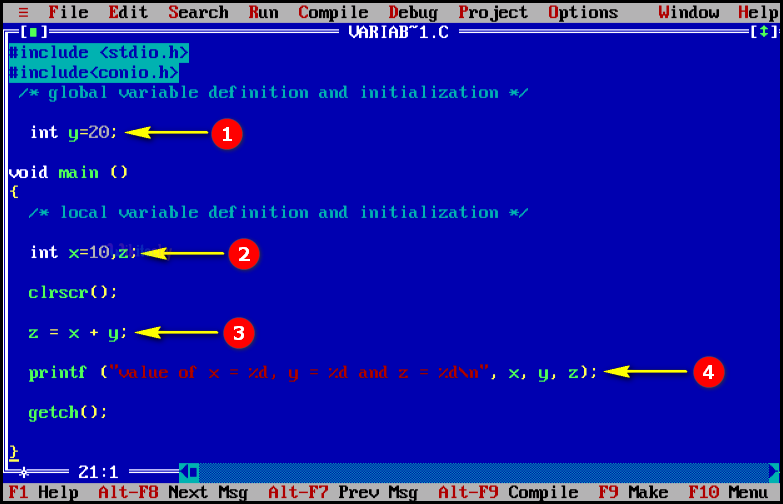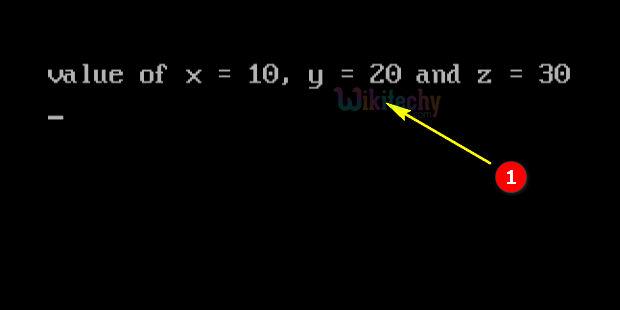# C - Variable ScopeLearn C - C tutorial - Variable scope - C examples - C programs

## C - Variable Scope - Definition and Usage

• In C- Programming when you define a variable inside the function block, it will be considered as local variable, which can be accessed only inside the function block.
• In C- Programming when you defining a variable outside the function block, it will be considered as global variable which can be accessed only outside the function block.## C Code - Explanation :1. Here in this statement we declare and assign the value for the variable “y” as a global variable and their datatype as integer.
2. In this statement we declare the variable and initialize the value of “x=10” & “z” as integer datatype.
3. In this statement we are adding value of the variable “x” and “y” and the output value will be stored in the variable “z”.
4. Here in this printf statement we print the value of x,y and z.

## Sample Output - Programming Examples1. Here in this output the variable “x” has been declared as global variable with its value “10” and in terms of “y” it was declared as local variable with its value “20” and the sum of the output value as “30” was stored in the variable “z” as shown in the console window.